PhysiologyWeb  Home  |  FAQ  |  About  |  Contact  |   - Sign InResting Membrane Potential -
Chord Conductance Equation
In this section, we will show how the chord conductance equation can be derived based on the membrane ionic current equations learned in the previous section. Very simply, the chord conductance equation can be used to determine the membrane potential based on the conductance values as well as equilibrium potential values of the ions contributing to the membrane potential. The derivation shown below assumes that a cell has ion channels for K+, Na+, and Cl and that these channels are open.
Previously in this lecture, we described in detail how the membrane potential is established and the factors that govern the value of the membrane potential. Briefly, if there is only one type of ion channel in the plasma membrane and those channels are open, the resting membrane potential will be at the equilibrium potential for that ion. For example, if there are only K+ channels in a cell plasma membrane, then Vm in this cell will be the same as the K+ equilibrium potential (VK), which can be obtained from the Nernst potential for K+ (see Nernst potential calculator). Therefore, in this cell, Vm = Vrest = VK, which is what you expect because K+ distributes itself across the membrane according to the Nernst equation. As you recall, the Nernst equation describes the situation at equilibrium for one ion (see Nernst Equilibrium Potential). Of course, in this example, the K+ channels have to be open to allow K+ to redistribute itself across the membrane according to the chemical and electrical potential gradients described previously. At VK, there is no net movement of K+ across the membrane. Since in solutions, electrical current is carried by ions, in this cell at VK, the net current carried by K+ across the membrane is zero (i.e., IK = 0). Thus, at equilibrium, the net current across the membrane is zero. Remember from the previous section that Ohm's law can be used to obtain the current carried by an ion across the plasma membrane according to Equation 1: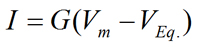Eq. 1
where I is current carried by an ion (in Amperes), G is conductance for the ion (in Siemens), Vm is the membrane potential (in Volts), and VEq. is the equilibrium potential for the ion (in Volts). Recall that the difference between Vm and VEq. is the driving force (DF) that acts on the ion (DF = VmVEq.). The direction of ion movement across the plasma membrane will depend on the ionic species and on the arithmetic sign (i.e., positive or negative) of the driving force. The direction of current flow (carried by the ionic species) will depend on the charge carried by the ion (see Table in the previous section). The magnitude of the current carried by the ionic species will depend on the magnitude of the driving force as well as the magnitude of conductance for that ion.
Similarly, if there are only Na+ channels in the cell (and are open), at equilibrium, Vm = Vrest = VNa. And at equilibrium, INa = 0. In the same fashion, if there are only Cl channels in the cell (and are open), at equilibrium, Vm = Vrest = VCl. And at equilibrium, ICl = 0. These principles were described fully previously in this lecture (see Nernst Equilibrium Potential).
If there are more than one type of ion channels in the cell plasma membrane and the channels are open, all participating ions contribute to the establishment of the membrane potential. In this case, the value of the membrane potential can be determined by using the Goldman-Hodgkin-Katz equation (GHK equation). Now, the resting potential can no longer be described as an equilibrium potential because no ion is at equilibrium (Vm = VrestVEq.). Rather, each ion moves down its own electrochemical potential gradient. Because the membrane potential is not at the equilibrium potential for any ion, there is a driving force that acts on each ion according to DF = VmVEq., causing the ion to move into or out of the cell depending on the direction (i.e., arithmetic sign) of the driving force. The system as a whole, however, is said to be at steady-state because the resting membrane potential remains stable (so long as no perturbation is applied to the system).
At steady-state, the resting membrane potential is stable (i.e., not changing), however, each of the ions contributing to the membrane potential moves down its own electrochemical potential gradient according to Equation 1. Assuming that a cell plasma membrane has channels only for K+, Na+, and Cl and these channels are open (i.e., GK ≠ 0, GNa ≠ 0, and GCl ≠ 0), the following current equations can be written for K+, Na+, and Cl: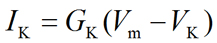Eq. 2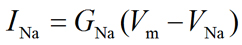Eq. 3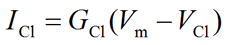Eq. 4
where IK, INa, and ICl are the current values resulting from transmembrane movement of K+, Na+, and Cl, respectively. GK, GNa, and GCl are the ion conductance values for each ion. VK, VNa, and VCl are the equilibrium potential values for each ion. Finally, Vm is the membrane potential.
Based on the driving force and the nature of ionic species (cation or anion), each ion may move into or out of the cell. As each ion moves down its own electrochemical gradient across the plasma membrane, it leads to the generation of a transmembrane current that may be described by Equations 2 through 4 shown above. As noted above, the arithmetic sign of the current is determined by the arithmetic sign of the driving force. Therefore, in order to determine the total current (or net current) moving across the plasma membrane (Im), we must add the individual currents resulting from the transmembrane movement of each ion. Thus, the total membrane current (Im) may be expressed as the algebraic sum of all the individual ion currents (Equation 5).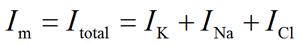Eq. 5
where Im and Itotal represent the total or net current moving across the plasma membrane, and IK, INa, and ICl are the individual current values resulting from the transmembrane movement of K+, Na+, and Cl, respectively.
Since at steady-state, the membrane potential is not changing, it follows that at steady-state, the net movement of ions across the plasma membrane is zero. By extension, at steady-state the net current across the membrane is zero (Im = 0). Therefore, at the steady-state, the following will be true (Equation 6):Eq. 6
Replacing IK, INa, and ICl in Equation 6 with corresponding terms from Equations 2 through 4 will yield Equation 7: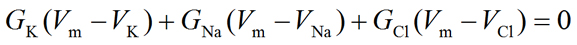Eq. 7
Expanding Equation 7, we will obtain Equation 8: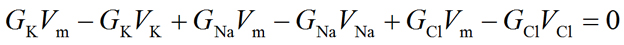Eq. 8
Rearranging Equation 8 will yield Equation 9: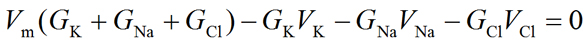Eq. 9
Further rearrangement of Equation 9 will yield Equation 10:Eq. 10
Finally, solving for Vm will yield the chord conductance equation (Equation 11):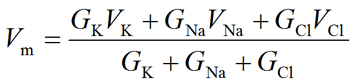Eq. 11
The chord conductance equation is useful in many ways. The membrane potential is described as a weighted average of the equilibrium potentials, and the magnitude of the conductance for all the ions contributing to the membrane potential. The magnitude of the conductance for each ion is related to total number of open channels for that ion, and determines the contribution of that ion to Vm. The larger the conductance of a given ion, the larger the contribution of that ion will be in setting the membrane potential. For example, it can be seen that if GK is very large (compared with GNa and GCl), Vm will be closer to VK than it will be to VNa or VCl. Notice that if the channels for a given ion are all closed (i.e., the conductance for that ion is zero), the above expression is reduced and simplified to include only the terms for the other two ions. Remember that the current carried by each ion will tend to move the membrane potential towards the equilibrium potential for that ion. Thus, if GK is much larger than GNa and GCl, then Vm will be closer to VK, although it will never be exactly at VK unless both GNa = 0 and GCl = 0. Of course, if both GNa = 0 and GCl = 0, then it can be seen that Equation 11 will be reduced to simply Vm = VK. In this case, K+ is the only ion that contributes to the establishment of the membrane potential and, hence, Vm will be exactly equal to VK as predicted by the Nernst equation.
A close examination of the chord conductance equation also reveals that this equation shares a similarity with the Goldman-Hodgkin-Katz (GHK) equation. Both equations rely on the total number of open channels for the ions in the system to determine the resting membrane potential. In the chord conductance equation, the conductance for each ion is used as a major determinant of that ion's contribution to the membrane potential. In the Goldman-Hodgkin-Katz equation, the relative permeability for each ion is used as a major determinant of that ion's contribution to the membrane potential. Both conductance and permeability are related to the total number of open channels in the plasma membrane. In fact, conductance is the electrical equivalent of membrane permeability for an ion.
 Previous: Membrane Ionic Current Equations Next: Gibbs-Donnan Equilibrium

Posted: Saturday, February 15, 2014
Last updated: Friday, November 24, 2017